• 作者：張張張張 ...【转载请注明出处，谢谢！】 一、决策树概述 决策树（Decision Tree）算法是一种基本的分类与回归方法，是最...构造决策树的基本思想是随着树深度的增加，节点的“熵”迅速降低，熵降低的速度越快越...
作者：張張張張
github地址：https://github.com/zhanghekai
【转载请注明出处，谢谢！】
【机器学习系列】之“西瓜数据集”决策树构建数学公式计算过程
【机器学习系列】之决策树剪枝和连续值、缺失值处理数学公式计算
【机器学习系列】之ID3、C4.5、CART决策树构建代码
文章目录一、决策树概述二、决策树场景三、决策树概念须知1.名词定义2.构建“树”时的基本要求四、三种决策树对比五、 划分选择1.ID3信息增益2.C4.5信息增益率3.CART基尼系数
一、决策树概述
决策树（Decision Tree）算法是一种基本的分类与回归方法，是最经常使用的数据挖掘算法之一。决策树是一种非线性有监督分类模型必须将已有的数据进行离散化，即：从字符串变成数值。构造决策树的基本思想是随着树深度的增加，节点的“熵”迅速降低，熵降低的速度越快越好，这样我们有望得到一棵高度最矮的决策树。
决策树模型呈树形结构，在分类问题中，表示基于特征对实例进行分类的过程。它可以认为是 if-then 规则的集合，也可以认为是定义在特征空间与类空间上的条件概率分布。
决策树的定义：分类决策树模型是一种描述对实例进行分类的树形结构。决策树由结点（node）和有向边（directed edge）组成。结点有两种类型：内部结点（internal node）和叶结点（leaf node）。内部结点表示一个特征或属性(features)，叶结点表示一个类(labels)。
用决策树对需要测试的实例进行分类：从根节点开始，对实例的某一特征进行测试，根据测试结果，将实例分配到其子结点；这时，每一个子结点对应着该特征的一个取值。如此递归地对实例进行测试并分配，直至达到叶结点。最后将实例分配到叶结点的类中。
决策树学习通常包括 3 个步骤：特征选择、决策树的生成和决策树的修剪。
二、决策树场景

一个叫做 “二十个问题” 的游戏，游戏的规则很简单：参与游戏的一方在脑海中想某个事物，其他参与者向他提问，只允许提 20 个问题，问题的答案也只能用对或错回答。问问题的人通过推断分解，逐步缩小待猜测事物的范围，最后得到游戏的答案。

一个邮件分类系统，大致工作流程如下：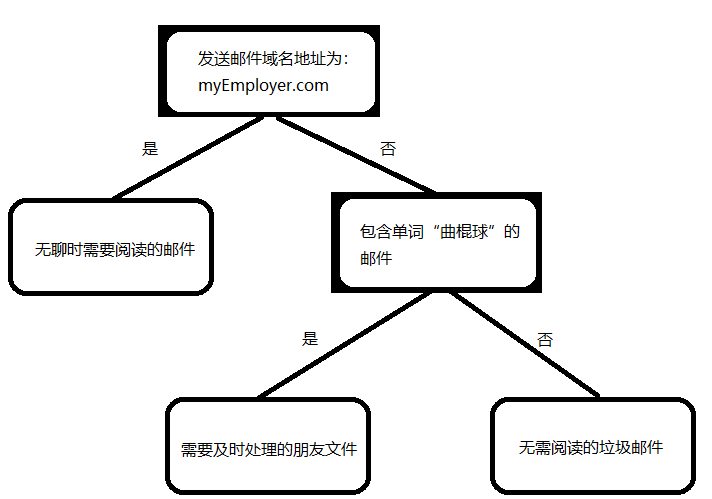首先检测发送邮件域名地址。如果地址为 myEmployer.com, 则将其放在分类 "无聊时需要阅读的邮件"中。
如果邮件不是来自这个域名，则检测邮件内容里是否包含单词 “曲棍球” , 如果包含则将邮件归类到 “需要及时处理的朋友邮件”, 如果不包含则将邮件归类到 “无需阅读的垃圾邮件” 。

三、决策树概念须知
1.名词定义
熵（entropy）：指体系的混乱的程度，在不同的学科中也有引申出的更为具体的定义，是各领域十分重要的参量。
信息熵（information theory）：使度量样本集合纯度最常用的一种指标。信息熵度量了事物的不确定性，越不确定的事物，它的熵就越大。
信息增益（information gain）：在划分数据集前后信息熵发生的变化称为信息增益。信息增益越大，表明数据“纯度”提升越大。
信息增益率（infor gain ratio）：正信息增益的基础上，解决过拟合问题的方法。
基尼系数（Gini index）：CART决策树划分属性的指标，数据集的纯度可以用基尼值来度量，基尼值越小，数据集的纯度越高。
纯度（purity）：叶子节点中正确分类的标签所占该叶子节点中包含数据的比例。
2.构建“树”时的基本要求

决策树的生成是一个递归过程，即决策树以深度优先遍历进行构建。
每个节点可选择的特征为：除该节点的父节点和祖父节点之外的所有特征。
若当前节点为空集，对应的处理措施为：将其设置为叶节点，类别设置为其父节点，所含样本最多的类别。

四、三种决策树对比

支持模型
树结构
特征选择
连续值处理

ID3
分类
多叉树
信息增益
不支持

C4.5
分类
多叉树
信息增益率
支持

CART
分类、多回归
二叉树
基尼系数、均方差
支持

五、 划分选择
我们希望决策树的分支节点所包含的样本尽可能属于同一类别，即节点的“纯度”越来越高。
我们使用周志华一书《机器学习》中的“西瓜数据集”进行计算：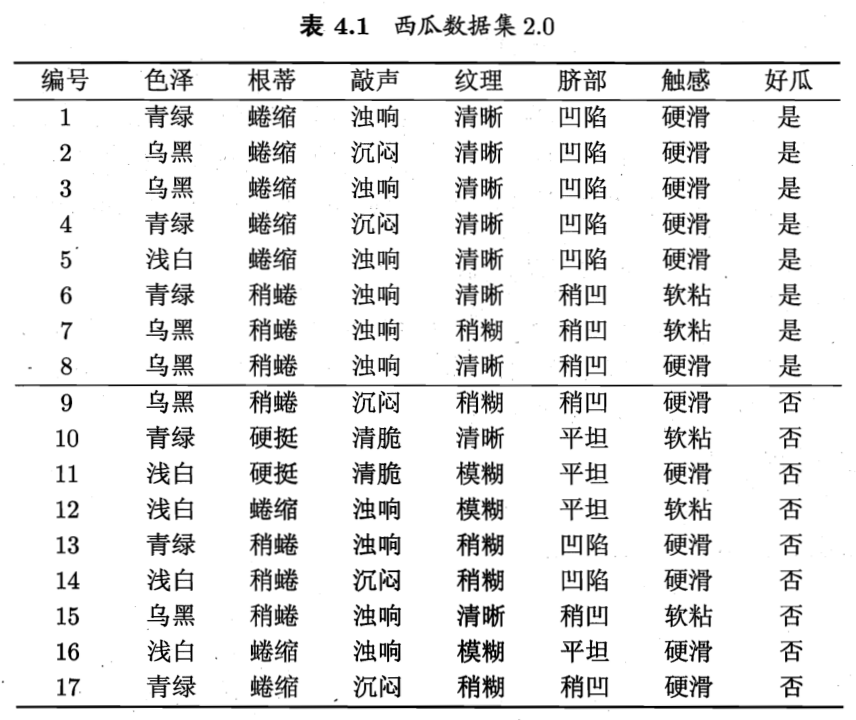1.ID3信息增益
$信息熵：Ent(D) = - \sum_{k = 1}^{|Y|}p_k log_2 p_k$
$信息增益：Gain(D,a) = Ent(D) - \sum_{v = 1}^{V}\frac{|D^v|}{|D|}Ent(D^v)$

|Y|：代表分类标签的数量，“好瓜”、“坏瓜”，所以|Y|=2
a:代表数据集所包含的特征，a={色泽，根蒂，敲声，纹理，脐部，触感}
v：代表每一个特征下所包含的属性，例如特征“色泽“下v={青绿，乌黑，浅白}
正例：”是“好瓜
反例：”否“好瓜
$\frac{|D^v|}{|D|}$：代表该节点所包含的数据数量占其父节点数据数量的比例

根节点包含D中所有数据，数据总数：17，正例占p1=8/17，反例占p2=9/17。
根节点的信息熵为：
$Ent(D)=-\sum_{k=1}^{2}p_k log_2 p_k=-(\frac{8}{17}log_2 \frac{8}{17}+\frac{9}{17}log_2 \frac{9}{17})=0.998$

​	然后，我们要计算出当前特征集合{色泽，根蒂，敲声，纹理，脐部，触感}中每个特征的信息熵和信息增益。
★特征”色泽“：
​		$D^1$（色泽=青绿）：{1，4，6，10，13，17}，正例p1=3/6，反例p2=3/6
​		$Ent(D^1)=-(\frac{3}{6}log_2 \frac{3}{6} + \frac{3}{6}log_2 \frac{3}{6}) = 1.000$
​		$D^2$（色泽=乌黑）：{2，3，7，8，9，15}，正例p1=4/6，反例p2=2/6
​		$Ent(D^2)=-(\frac{4}{6}log_2 \frac{4}{6} + \frac{2}{6}log_2 \frac{2}{6}) = 0.918$
​		$D^3$（色泽=浅白）：{5，11，12，14，16}，正例p1=1/5，反例p2=4/5
​		$Ent(D^3)=-(\frac{1}{5}log_2 \frac{1}{5} + \frac{4}{5}log_2 \frac{4}{5}) = 0.722$
"色泽"特征的信息增益：
$Gain(D,色泽)=Ent(D) - \sum_{v = 1}^{V}\frac{|D^v|}{|D|}Ent(D^v)\\ =0.998-(\frac{6}{17}\times 1 +\frac{6}{17}\times 0.918 + \frac{5}{17}\times 0.722)=0.109$
类似的，计算出其他特征的信息增益：
Gain（D，根蒂）=0.143		$\quad$ Gain（D，敲声）=0.141	$\quad$ 	Gain（D，纹理）=0.381
Gain（D，脐部）=0.289		$\quad$ Gain（D，触感）=0.006
特征”纹理”的信息增益最大，选他作为划分属性。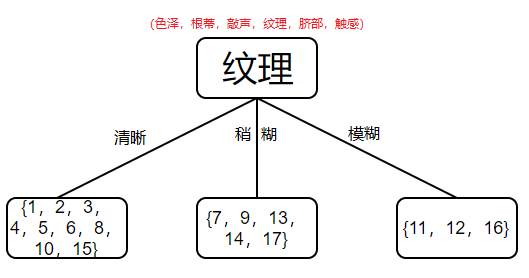其中节点上方红色字体表示：创建该node节点时可选择的特征。

$D^1$中有编号为{1，2，3，4，5，6，8，10，15}，可用特征集合为{色泽，根蒂，敲声，脐部，触感}，其中正例p1=7/9，反例p2=2/9。

$D^1$节点的信息熵为：
$Ent(D^1)=-\sum_{k=1}^{2}p_k log_2 p_k=-(\frac{7}{9}log_2 \frac{7}{9}+\frac{2}{9}log_2 \frac{2}{9})=0.763$
★特征”色泽“：
​		$D^{11}$（色泽=青绿）：{1，4，6，10}，正例p1=3/4，反例p2=1/4
​		$Ent(D^{11})=-(\frac{3}{4}log_2 \frac{3}{4} + \frac{1}{4}log_2 \frac{1}{4}) = 0.811$
​		$D^{12}$（色泽=乌黑）：{2，3，8，15}，正例p1=3/4，反例p2=1/4
​		$Ent(D^{12})=-(\frac{3}{4}log_2 \frac{3}{4} + \frac{1}{4}log_2 \frac{1}{4}) = 0.811$
​		$D^{13}$（色泽=浅白）：{5}，正例p1=1，反例p2=0
​		$Ent(D^{13})=-(1log_2 1 + 0log_2 0) =0$
"色泽"特征的信息增益：
$Gain(D^1,色泽)=Ent(D^1) - \sum_{v = 1}^{V}\frac{|D^v|}{|D|}Ent(D^v)\\ =0.763-(\frac{4}{9}\times 0.811 +\frac{4}{9}\times 0.811 + \frac{1}{9}\times 0)=0.043$
类似的，计算出其他特征的信息增益：
Gain（D1，根蒂）=0.458	$\quad$ 	Gain（D1，敲声）=0.331
Gain（D1，脐部）=0.458	$\quad$ 	Gain（D1，触感）=0.458
其中“根蒂”，“脐部”，“触感”均获得最大信息增益，可任选其一作为特征划分。此处我们选择“根蒂”特征。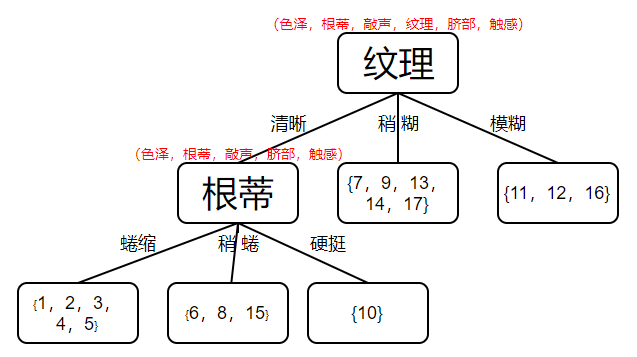$D^{11}$中有编号为{1，2，3，4，5}，可用特征集合为{色泽，敲声，脐部，触感}，其中正例p1=1，反例p2=0。
$D^{11}$节点的信息熵为：
$Ent(D^{11})=-\sum_{k=1}^{2}p_k log_2 p_k=-(1log_2 1 +0log_2 0)=0$

由于Ent（D11）的值已达最小，说明D11中数据纯度已达到最高，数据分类已完全分类，则无需再往下进行划分，将该节点设置为叶子节点。

$D^{12}$中编号为{6，8，15}，可用特征集合为{色泽，敲声，脐部，触感}，其中正例p1=2/3，反例p2=1/3。
$D^{12}$节点的信息熵为：
$Ent(D^{12})=-\sum_{k=1}^{2}p_k log_2 p_k=-(\frac{2}{3}log_2 \frac{2}{3} +\frac{1}{3}log_2 \frac{1}{3})=0.918$
★特征“色泽”：
​		$D^{121}$（色泽=青绿）：{6}，正例p1=1，反例p2=0
​		$Ent(D^{121})=-(1log_2 1 + 0log_2 0) = 0$
$D^{122}$（色泽=乌黑）：{8，15}，正例p1=1/2，反例p2=1/2
​		$Ent(D^{122})=-(\frac{1}{2}log_2 \frac{1}{2} + \frac{1}{2}log_2 \frac{1}{2}) = 1$
​		$D^{123}$（色泽=浅白）：{ }，正例p1=0，反例p2=0
​		$Ent(D^{123})=-(0log_2 0 + 0log_2 0) =0$

"色泽"特征的信息增益：
$Gain(D^{12},色泽)=Ent(D^{12}) - \sum_{v = 1}^{V}\frac{|D^v|}{|D|}Ent(D^v)\\ =0.918-(\frac{1}{3}\times 0 +\frac{2}{3}\times 1 + 0\times 0)=0.251$
类似的，计算出其他特征的信息增益：
Gain（D12，敲声）=0	$\quad$ 	Gain（D12，脐部）=0$\quad$ 	Gain（D12，触感）=0.251
其中“色泽”和“触感”均获得最大信息增益，可任选其一作为特征划分。此处我们选择“色泽”特征。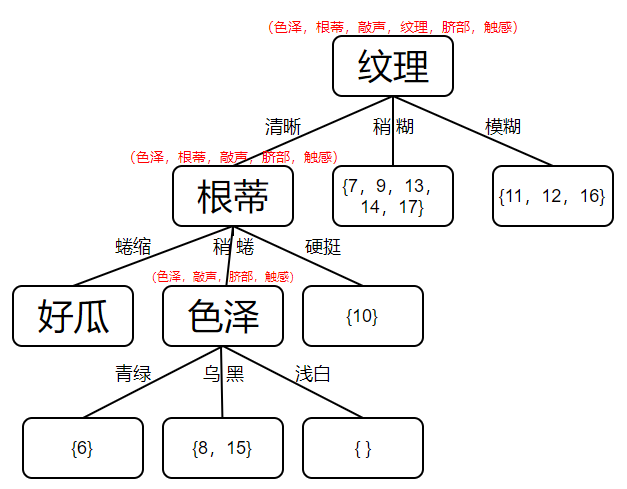$D^{121}$中编号为{6}，可用特征集合为{敲声，脐部，触感}，其中p1=1，p2=0。
$D^{121}$节点的信息熵为：
$Ent(D^{121})=-\sum_{k=1}^{2}p_k log_2 p_k=-(1log_2 1 +0 log_2 0)=0$
将该节点划分为叶子节点。

$D{122}$中编号为{8，15}，可用特征集合为{敲声，脐部，触感}，p1=1/2，p2=1/2。
$D^{122}$节点的信息熵为：
$Ent(D^{122})=-\sum_{k=1}^{2}p_k log_2 p_k=-(\frac{1}{2}log_2 \frac{1}{2} +\frac{1}{2} log_2 \frac{1}{2})=1$
★特征“触感”：
​		$D^{1221}$（触感=硬滑）：{8}，正例p1=1，反例p2=0
​		$Ent(D^{1221})=-(1log_2 1 + 0log_2 0) = 0$
​		$D^{1222}$（触感=软粘）：{15}，正例p1=0，反例p2=1
​		$Ent(D^{1221})=-(0log_2 0 + 1log_2 1) = 0$
"触感"特征的信息增益：

$Gain(D^{122},色泽)=Ent(D^{122}) - \sum_{v = 1}^{V}\frac{|D^v|}{|D|}Ent(D^v)\\ =1-(\frac{1}{2}\times 0 +\frac{1}{2}\times 0)=1$
类似的，计算出其他特征的信息增益：
Gain（D122，敲声）=0	$\quad$ 	Gain（D122，脐部）=0
所以选择“触感”特征作为划分节点。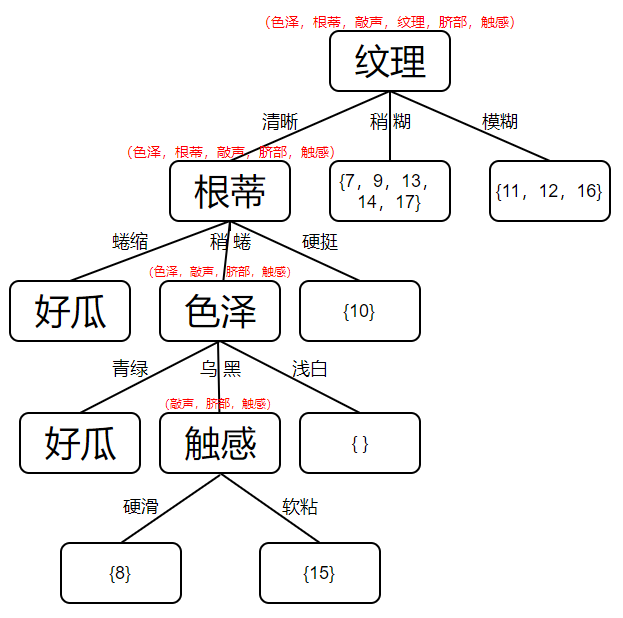$D^{1221}$中编号为{8}，可用特征集合为{敲声，脐部}，p1=1，p2=0。
$D^{1221}$节点的信息熵为：
$Ent(D^{1221})=-\sum_{k=1}^{2}p_k log_2 p_k=-(1log_2 1 +0 log_2 0)=0$
所以将该节点设置为叶子节点。

$D^{1222}$中编号为{15}，可用特征集合为{敲声，脐部}，p1=0，p2=1。
$D^{1222}$节点的信息熵为：
$Ent(D^{1222})=-\sum_{k=1}^{2}p_k log_2 p_k=-(0log_2 0 +1 log_2 1)=0$

此时需要往回遍历，找到第三层“色泽”特征下的D123属性集合。

$D^{123}$中为空集{ }，将其设置为叶节点，且类别设置为其父节点所含样本最多的类别即{6，8，15}
中，p1=2/3，p2=1/3，所以该叶子节点类别为“好瓜”。

继续往回遍历，找到第二层“根蒂”特征下的D13属性集合。

$D^{13}$中编号为{10}，可用特征集合为{色泽，敲声，脐部，触感}，p1=0，p2=1。
$D^{13}$节点的信息熵为：
$Ent(D^{13})=-\sum_{k=1}^{2}p_k log_2 p_k=-(0log_2 0 +1 log_2 1)=0$
所以将该节点设置为叶子节点。

继续往回遍历，找到第一层“纹理”特征下的D2属性集合。

$D^{2}$中编号为{7，9，13，14，17}，可用特征集合为{色泽，根蒂，敲声，脐部，触感}，p1=1/5，p2=4/5。
$D^{2}$节点的信息熵为：
$Ent(D^{2})=-\sum_{k=1}^{2}p_k log_2 p_k=-(\frac{1}{5}log_2 \frac{1}{5} +\frac{4}{5} log_2 \frac{4}{5})=0.722$
★特征“色泽”：
​		$D^{21}$（色泽=青绿）：{13，17}，正例p1=0，反例p2=1
​		$Ent(D^{21})=-(0log_2 1 + 1log_2 0) = 0$
​		$D^{22}$（色泽=乌黑）：{7，9}，正例p1=1/2，反例p2=1/2
​		$Ent(D^{22})=-(\frac{1}{2}log_2 \frac{1}{2} + \frac{1}{2}log_2 \frac{1}{2}) = 1$
​		$D^{23}$（色泽=浅白）：{14}，正例p1=0，反例p2=1
​		$Ent(D^{23})=-(0log_2 0 + 1log_2 1) =0$
"色泽"特征的信息增益：

$Gain(D^{2},色泽)=Ent(D^{2}) - \sum_{v = 1}^{V}\frac{|D^v|}{|D|}Ent(D^v)\\ =0.722-(\frac{2}{5}\times 0 +\frac{2}{5}\times 1 + \frac{1}{5}\times 0)=0.322$
类似的，计算出其他特征的信息增益：
Gain（D2，敲声）=0.322	$\quad$ 	Gain（D2，脐部）= 0.172
Gain（D2，触感）= 0.722    $\quad$   Gain（D2，根蒂）= 0.073
特征“触感”的信息增益最大，选他作为划分属性。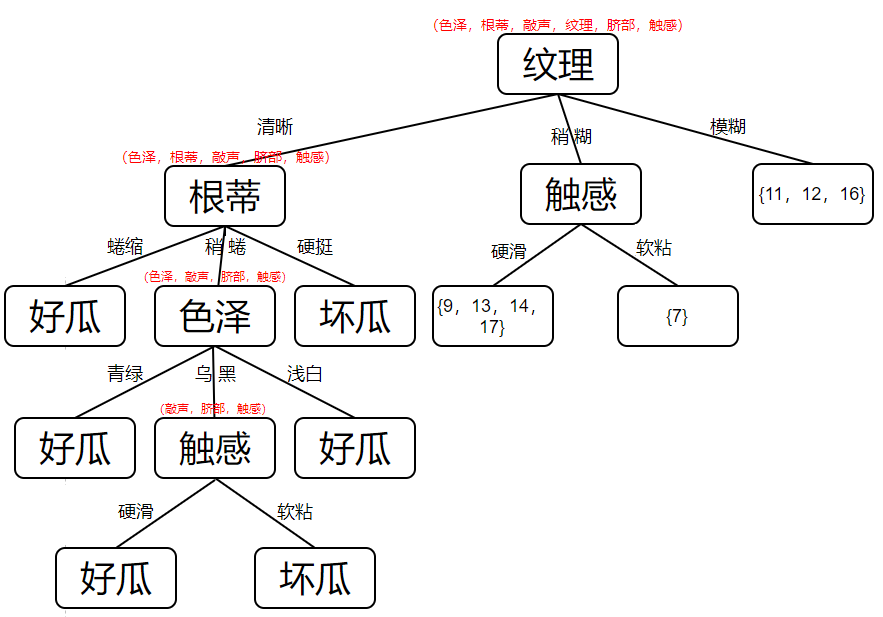12. $D^{21}$中编号为{9，13，14，17}，可用特征集合为{色泽，根蒂，敲声，脐部}，其中正例p1=0，反例p2=1.
$D{21}$节点的信息熵为：
$Ent(D^{21})=-\sum_{k=1}^{2}p_k log_2 p_k=-(0log_2 0 +1 log_2 1)=0$
所以将该节点划分为叶子节点。

$D^{22}$中编号为{7}，可用特征集合为{色泽，根蒂，敲声，脐部}，其中正例p1=1，反例p2=0.

$D{22}$节点的信息熵为：
$Ent(D^{22})=-\sum_{k=1}^{2}p_k log_2 p_k=-(1log_2 1 +0 log_2 0)=0$
所以将该节点划分为叶子节点。

往回遍历，找到第一层“纹理”特征下的D3属性集合。

$D^3$中有编号为{11，12，16}，可用特征集合为{色泽，根蒂，敲声，脐部，触感},其中正例p1=0，反例p2=1。

$D{3}$节点的信息熵为：
$Ent(D^{3})=-\sum_{k=1}^{2}p_k log_2 p_k=-(0log_2 0 +1 log_2 1)=0$
所以将其设置为叶子节点。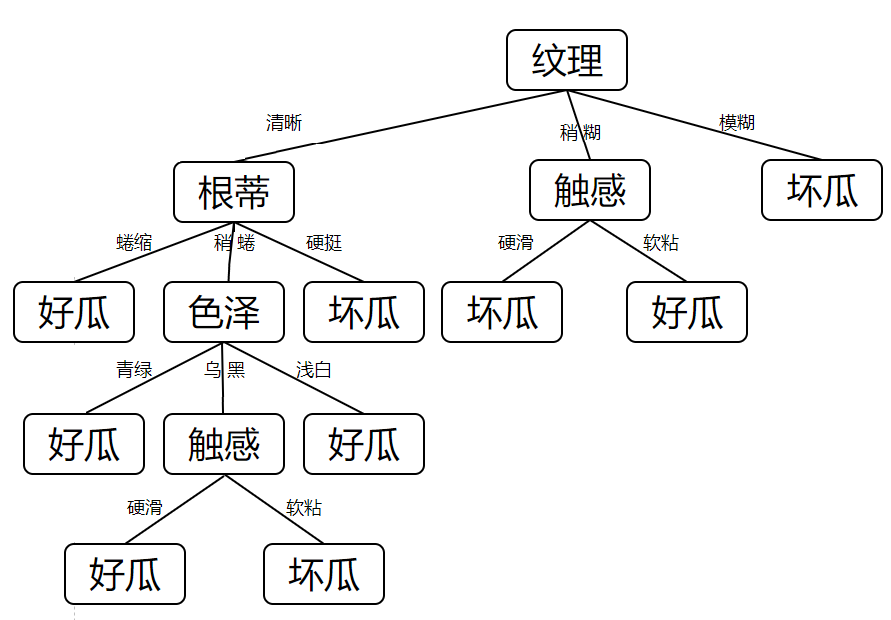至此，使用信息增益构建的ID3决策树已经建立好了，如上图所示。
2.C4.5信息增益率
信息增益缺点：是对可取属性多的特征有偏好，比如如果把“编号”这一列当作特征也考虑在内，那么可以计算处它的信息增益大于其他的候选特征，因为“编号”有17个可取的数值，产生17个分支，每个分支节点仅包含一个样本，显然这些分支节点的纯度最大。但是，这样的决策树显然不具有泛化能力，无法对新样本进行有效预测。C4.5决策树算法：使用“信息增益率”来选择最优划分属性，可以很好的克服上述缺点。
信息增益率定义为：
$Gain_ratio(D,a)=\frac{Gain(D,a)}{IV(a)}$
其中：
$IV(a)=-\sum_{v=1}^{V} \frac{|D^v|}{|D|}log_2 \frac{|D^v|}{|D|}IV(a)$
IV(a)称为特征a的“固有值”，特征a的可能取值数目越多（即V越大），则 IV(a)的值通常会越大。但增益率也可能产生一个问题就是对属性较少的特征有所偏好。注意：C4.5算法并不是直接选择增益率最大的候选划分特征，而是先从候选划分特征中找出信息增益高于平均水平的特征，再从中选择增益率最高的。

由于信息增益的计算方法在ID3中已经详细介绍并给出计算例子，在这里不再赘述，重点计算信息增益率的求解。

计算数据集D中所有特征的信息增益和信息增益率：
Gain(D,色泽 ) = 0.109	$\quad$ 	Gain(D,根蒂) = 0.143	$\quad$ 	Gain(D,敲声) = 0.141
Gain(D,纹理) = 0.381	$\quad$ 	Gain(D,脐部) = 0.289	$\quad$ 	Gain(D,触感) = 0.006
$ave\_Gain(D)=\frac{0.109+0.143+0.141+0.381+0.289+0.006}{6}=0.178$
选择信息增益高于平均水平的特征，即选择“纹理”和“脐部”计算信息增益率：
★特征“纹理”：清晰：9；稍糊：5；模糊：3
$IV(纹理)=-(\frac{9}{17}log_2 \frac{9}{17} + \frac{5}{17}log_2 \frac{5}{17} + \frac{3}{17}log_2 \frac{3}{17})=1.446$
信息增益率为：
$Gain\_ratio(D,纹理)=\frac{0.381}{1.446}=0.263$
★特征“脐部”：凹陷：7；稍凹：6；平坦：4
$IV(脐部)=-(\frac{7}{17}log_2 \frac{7}{17} + \frac{6}{17}log_2 \frac{6}{17} + \frac{4}{17}log_2 \frac{4}{17})=1.548$
信息增益率为：
$Gain\_ratio(D,脐部)=\frac{0.289}{1.548}=0.187$
“纹理”的信息增益率大于“脐部”的信息增益率，所以选择特征“纹理”当作节点，划分数据集。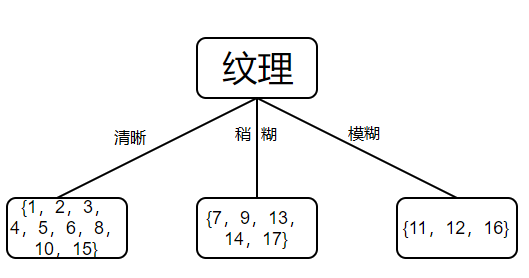计算数据集D1中可用特征的信息增益和信息增益率：
Gain(D1,色泽 ) = 0.043	$\quad$ 	Gain(D1,根蒂) = 0.458	$\quad$ 	Gain(D1,敲声) = 0.331
Gain(D1,脐部) = 0.458		$\quad$ Gain(D1,触感) = 0.458
$ave\_Gain(D1)=\frac{0.043+0.458+0.331+0.458+0.458}{5}=0.3496$
选择信息增益高于平均水平的特征，即选择“根蒂”、“触感”和“脐部”计算信息增益率：
★特征“根蒂”：蜷缩：5；稍蜷：3；硬挺：1
$IV(根蒂)=-(\frac{5}{9}log_2 \frac{5}{9} + \frac{3}{9}log_2 \frac{3}{9} + \frac{1}{9}log_2 \frac{1}{9})=1.351$
信息增益率为：
$Gain\_ratio(D1,根蒂)=\frac{0.458}{1.351}=0.339$
★特征“脐部”：凹陷：5；稍凹：3；平坦：1
$IV(脐部)=-(\frac{5}{9}log_2 \frac{5}{9} + \frac{3}{9}log_2 \frac{3}{9} + \frac{1}{9}log_2 \frac{1}{9})=1.351$
信息增益率为：
$Gain\_ratio(D1,脐部)=\frac{0.458}{1.351}=0.339$
★特征“触感”：硬滑：6；软粘：3
$IV(触感)=-(\frac{6}{9}log_2 \frac{6}{9} + \frac{3}{9}log_2 \frac{3}{9} )=0.918$
信息增益率为：
$Gain\_ratio(D1,触感)=\frac{0.458}{0.918}=0.499$
“触感”的信息增益率最大，所以选择特征“触感”当作节点，划分数据集。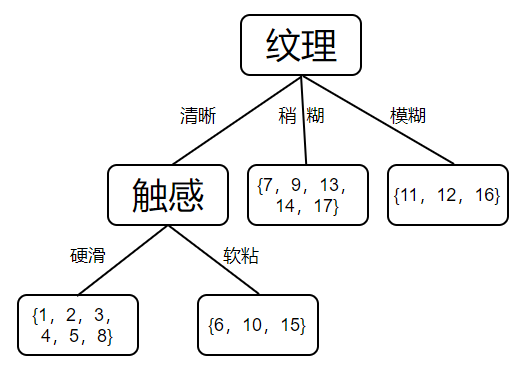计算数据集D11中的信息：
由于数据集D11的信息熵为0，所以此节点以完全分类，将其设置为叶子节点。

计算数据集D12中的信息：
Gain(D12,色泽)=0.251	$\quad$ 	Gain(D12,根蒂)=0.251
Gain(D12,敲声)=0.251		$\quad$ Gain(D12,脐部)=0.251
★特征“脐部”：凹陷：0；稍凹：2；平坦：1
$IV(脐部)=-(0 log_2 0 + \frac{2}{3}log_2 \frac{2}{3} + \frac{1}{3}log_2 \frac{1}{3})=0.251$
​	不难发现，这四个特征均是一个属性包含数据为0，一个属性包含数据为2，另一个属性包含数据为1，所以他们的信息增益率均相同，这种情况我们可以任选其一划分数据，类别为其中包含最多的类别；也可以将其设置为叶子节点，牺牲正确率换取更低的决策树层数。在这里我采取的方法为后者。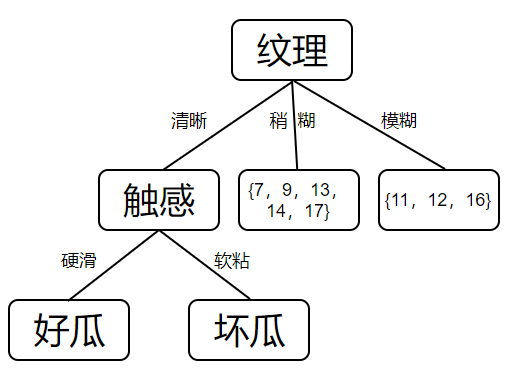计算数据集D2中的信息
类似于数据集D12的情况，由于D2中只包含一个正例，所以依然可以采取牺牲正确率换取更低的决策树层数，或是进行计算选出一个特征来划分。此处采取设置为叶节点，有兴趣的同学可以自行计算。

计算数据集D3中的信息
由于数据集D11的信息熵为0，所以此节点以完全分类，将其设置为叶子节点。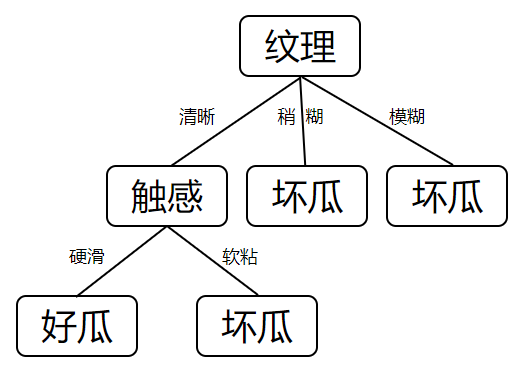3.CART基尼系数
CART决策树：使用”基尼指数“来选择划分特征。数据集的纯度可用基尼值(Gini)来度量。Gini(D)反映了从数据集D中随机抽取两个样本，其类别标记不一致的概率，Gini值越小，则数据集的纯度越高。
$Gini(D)=1-\sum_{k=1}^{|Y|}p_{k}^{2}$
特征的“基尼指数”(Gini index)定义如下，选择使得划分后基尼指数最小的特征作为最优划分特征。
$Gini\_index(D,a)=\sum_{v=1}^{V} \frac{|D^v|}{|D|}Gini(D^v)$
计算每个特征的基尼指数前，先计算下该节点的基尼值，若基尼值为0，则表示该节点下的数据集已完全分类。

根节点包含D中所有数据，数据总数为17，可用特征{色泽，根蒂，敲声，纹理，脐部，触感}：
★特征“色泽”：
青绿：{1，4，6，10，13，17}，数据总计：6，正例p1= 3/6，反例p2= 3/6
$Gini(青绿)=1-\sum_{k=1}^{|Y|}p_k^2=1-(\frac{3}{6}\times \frac{3}{6} + \frac{3}{6}\times \frac{3}{6})=0.5$
乌黑：{2，3，7，8，9，15}，数据总计：6，正例p1= 4/6，反例p2= 2/6
$Gini(乌黑)=1-\sum_{k=1}^{|Y|}p_k^2=1-(\frac{4}{6}\times \frac{4}{6} + \frac{2}{6}\times \frac{2}{6})=0.444$
浅白：{5，11，12，14，16}，数据总计：5，正例p1= 1/5，反例p2= 4/5
$Gini(浅白)=1-\sum_{k=1}^{|Y|}p_k^2=1-(\frac{1}{5}\times \frac{1}{5} + \frac{4}{5}\times \frac{4}{5})=0.32$
“色泽”特征的基尼指数为：
$Gini\_index(D,色泽)=\frac{6}{17}\times 0.5 +\frac{6}{17}\times 0.444 + \frac{5}{17} \times 0.32=0.427$
同理可以计算出其他特征的基尼指数为：
Gini_index(D,根蒂) = 0.422	$\quad$ 	Gini_index(D,敲声) = 0.424		$\quad$ Gini_index(D,纹理) = 0.277
Gini_index(D,脐部) = 0.345	$\quad$ 	Gini_index(D,触感) = 0.494
选择基尼指数最小的特征进行划分，所以此次划分使用“纹理”特征。D1中包含数据总数为9，可用特征{色泽，根蒂，敲声，脐部，触感}：
★特征“色泽”
青绿：{1，4，6，10}，数据总计：4，正例p1= 3/4，反例p2= 1/4
$Gini(青绿)=1-\sum_{k=1}^{|Y|}p_k^2=1-(\frac{3}{4}\times \frac{3}{4} + \frac{1}{4}\times \frac{1}{4})=0.375$
乌黑：{2，3，8，15}，数据总计：4，正例p1= 3/4，反例p2= 1/4
$Gini(乌黑)=1-\sum_{k=1}^{|Y|}p_k^2=1-(\frac{3}{4}\times \frac{3}{4} + \frac{1}{4}\times \frac{1}{4})=0.375$
浅白：{5}，数据总计：1，正例p1= 1，反例p2= 0
$Gini(浅白)=1-\sum_{k=1}^{|Y|}p_k^2=1-(1\times 1 + 0\times 0)=0$
“色泽”特征的基尼指数为：
$Gini\_index(D^1,色泽)=\frac{4}{9}\times 0.375 +\frac{4}{9}\times 0.375 + \frac{1}{9} \times 0=0.333$
同理可以计算出其他特征的基尼指数为：
Gini_index(D1,根蒂) = 0.148	$\quad$ 	Gini_index(D1,敲声) = 0.185
Gini_index(D1,脐部) = 0.148	$\quad$ 	Gini_index(D1,触感) = 0.148
由于“根蒂”、“脐部”和“触感”的基尼指数相同，任选其一作为特征进行划分数据集，这里我们选择“根蒂”特征：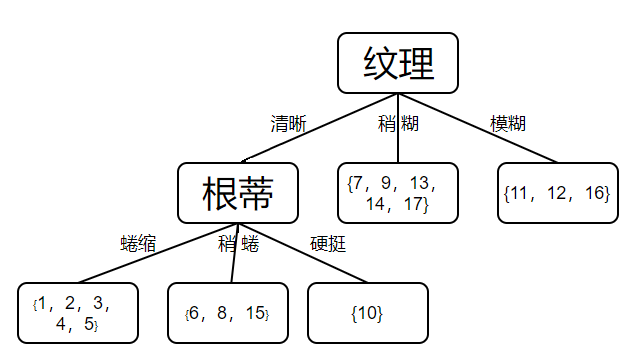D11中包含数据总数为5，其中正例p1=1，反例p2=0。
该节点基尼值为：
$Gini(D^11)=1-\sum_{k=1}^{|Y|}p_k^2=1-(1\times 1 +0 \times 0) = 0$
该节点基尼值已达最小，将其设置为叶子节点。

D12中包含数据总数位3，可用特征为{色泽，敲声，脐部，触感}
★特征“色泽”：
青绿：{6}，数据总计：1，正例p1= 1，反例p2= 1/40
$Gini(青绿)=1-\sum_{k=1}^{|Y|}p_k^2=1-(1\times 1 + 0\times 0)=0$
乌黑：{8，15}，数据总计：2，正例p1= 1/2，反例p2= 1/2
$Gini(乌黑)=1-\sum_{k=1}^{|Y|}p_k^2=1-(\frac{1}{2}\times \frac{1}{2} + \frac{1}{2}\times \frac{1}{2})=0.5$
浅白：{ }，数据总计：0，正例p1= 0，反例p2= 0
$Gini(浅白)=1-\sum_{k=1}^{|Y|}p_k^2=1-(0\times 0 + 0\times 0)=0$
“色泽”特征的基尼指数为：
$Gini\_index(D^{12},色泽)=\frac{1}{3}\times 0 +\frac{2}{3}\times 0.5 + 0 \times 0=0.333$
同理可以计算出其他特征的基尼指数为：
Gini_index(D12,敲声) = 0.444	$\quad$ 	Gini_index(D12,脐部) = 0.444	$\quad$ Gini_index(D12,触感) = 0.333
由于“色泽”和“触感”的基尼指数相同，任选其一作为特征进行划分数据集，这里我们选择“色泽”特征：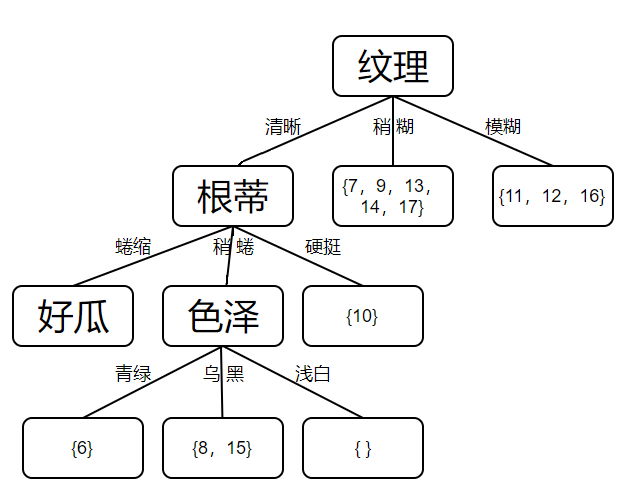D121中已完全分类，设置为叶节点。

D122中包含数据总数为2，可用特征为{敲声，脐部，触感}
Gini_index(D122,敲声) = 0.5	$\quad$ 	Gini_index(D122,脐部) = 0.5	$\quad$ 	Gini_index(D122,触感) = 0
所以选择“触感”特征划分数据集。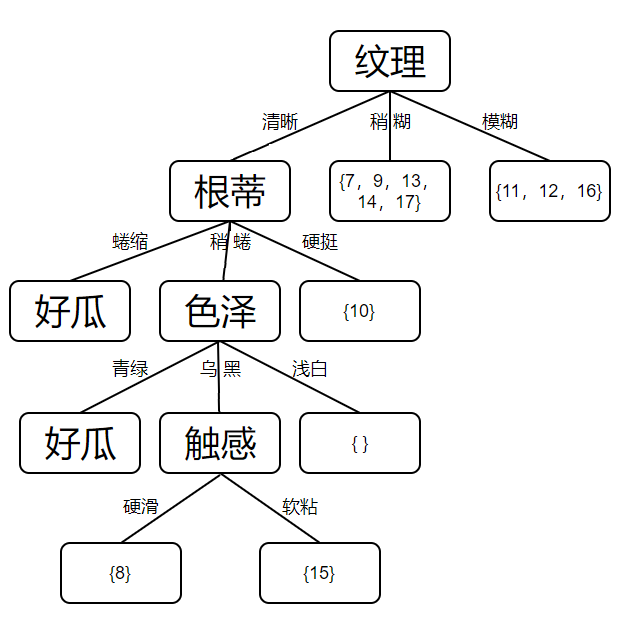D1221中已完全分类，设置为叶节点。

D1222中已完全分类，设置为叶节点。

往回遍历，找到第三层“色泽”特征下的D123属性集合。

该节点集合为空，设置为其父节点数据中类别最多的类。

往回遍历，找到第二层“根蒂”特征下的D13属性集合

D13中已完全分类，设置为叶节点。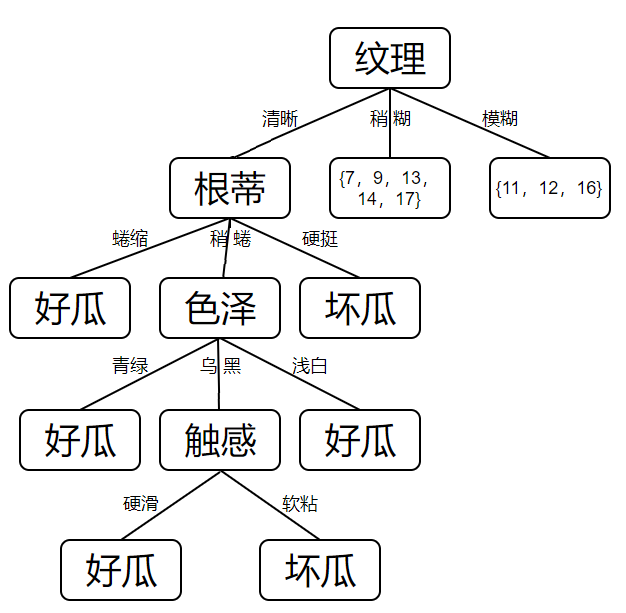往回遍历，找到第一层“纹理”特征下的D2属性集合

D2中包含数据总数为5，可用特征为{色泽，根蒂，敲声，脐部，触感}
Gini_index(D2,敲声) = 0.467		$\quad$ Gini_index(D2,脐部) = 0.267	$\quad$ 	Gini_index(D2,触感) = 0
Gini_index(D2,色泽) = 0.2	$\quad$ 	Gini_index(D1,根蒂) = 0.3
所以选择“触感”作为特征划分数据集。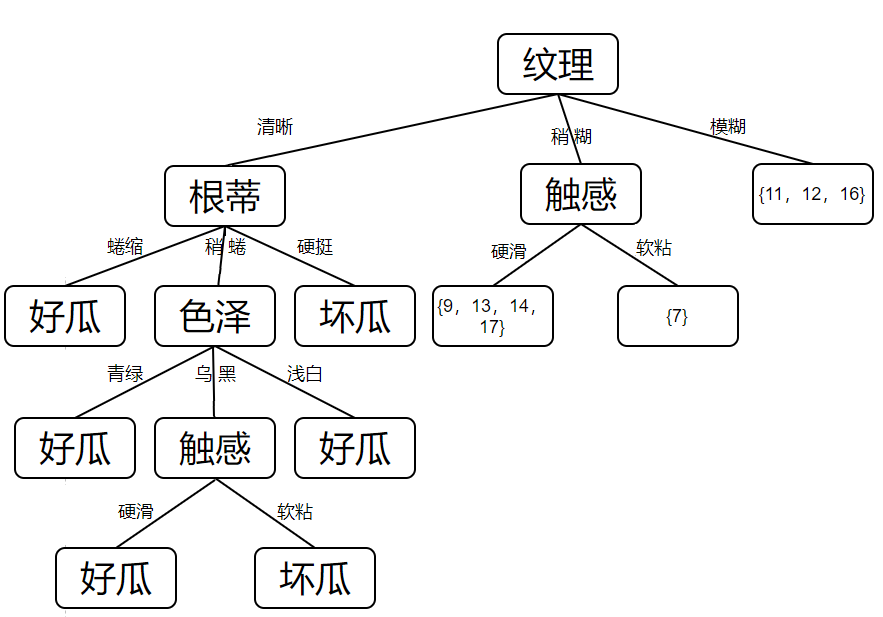D21中已完全分类，设置为叶节点。

D22中已完全分类，设置为叶节点。

D3中已完全分类，设置为叶节点。

建立好的决策树如下图所示：至此，三种决策树构建时的计算过程已经整理完了，在下一篇文章中我们来看看预剪枝与缺失值处理是怎么操作的。
【参考文献】

apache github主页：https://github.com/apachecn/AiLearning
天泽28 CSDN博客：https://blog.csdn.net/u012328159/article/details/70184415
刘建平 博客园：https://www.cnblogs.com/pinard/
周志华 《机器学习》


展开全文决策树 西瓜数据集 机器学习
• 根据瞬变电磁场"烟圈效应"理论,推导出全空间瞬变电磁视电阻率计算公式和由视电阻率-时间曲线计算视电阻率-深度曲线的数学模型,对矿井瞬变电磁探查技术进行了研究;研究出应用于井下探测巷道周围空间不同位置、不同...
• 如果存在多个池化层,就相当于网络中构造了一个多尺度特征金字塔,多尺度金字塔有利于提高检测/识别的稳定性 上述是池化层的优势,但是随着研究的深入,池化层的劣势也逐渐被发现,比如有实验发...
重点

根据不同类型,上采用上层反向传入的梯度信息

池化层反向传播公式推导

池化层在深度学习网络架构中的作用包括:
* 减少计算量
减少特征图尺寸,减少后面的层的计算量

提高多尺度信息
如果存在多个池化层,就相当于网络中构造了一个多尺度特征金字塔,多尺度金字塔有利于提高检测/识别的稳定性

上述是池化层的优势,但是随着研究的深入,池化层的劣势也逐渐被发现,比如有实验发现”均值池化不利于网络的收敛”,至于网络稳定性的问题,也有文献怀疑resnet这之类加深网络结构的优化会降低网络对目标位置误差的鲁棒性,池化带来的优势被抵消了不少,感觉是一个鸡肋般的层.

不论如何,池化层目前还是被广泛使用的,本文主要说明池化层如何进行反向误差传播的.

BP算法的具体推导可以参考BP反向传播公式推导,其中推导了梯度信息从k$k$$k$层传递到k−1$k-1$$k-1$层的公式如下:
δk−1i=δkj▽g(akj)wki${\delta }_{i}^{k-1}={\delta }_{j}^{k}▽g\left({a}_{j}^{k}\right){w}_{i}^{k}$
\delta _i^{k-1}=\delta _j^{k}\bigtriangledown g(a^k_j)w^k_i

池化层是没有激励函数的,可以认为激励函数g(x)=x$g\left(x\right)=x$$g(x)=x$,其导数▽g(x)$▽g\left(x\right)$$\bigtriangledown g(x)$=1.
池化层也没有可学习的权重w$w$$w$,可以认为w=1$w=1$$w=1$
所以上面的反向传播公式简化成
δk−1i=δkj${\delta }_{i}^{k-1}={\delta }_{j}^{k}$
\delta _i^{k-1}=\delta _j^{k}

似乎很简单,直接复制就可以了,但是池化层是会改变特征图尺寸的,所以上面等式两边的尺度不同,不能直接复制. 解决方法就是根据池化层类型,对梯度信息做上采样,令尺度相同.

均值池化
为了简单起见,假设池化层的kernel_size=strides$kernel\mathrm{_}size=strides$$kernel\_size=strides$.如果kernelsize=n$kerne{l}_{s}ize=n$$kernel_size=n$,则输出特征图中一个元素对应着n×n$n×n$$n \times n$个输入元素. 反向传播时,把反向传播进来的梯度平均分配给
n×n$n×n$$n \times n$个元素,达到上采样的目的
最大值池化
和均值池化类似,但是不是平均分配,而是直接复制给n×n$n×n$$n \times n$个元素中, 输入值最大的那个元素. 具体实现时,forward()时可以记录下max pooling取最大值的元素位置,反向时直接根据这个记录进行复制

展开全文池化层
• 在开始的少量迭代中利用K-FAC算法计算, 在后续迭代中构造秩–1矩阵, 通过Sherman-Morrison公式进行计算, 大大降低了计算复杂度. 实验结果表明, 改进的K-FAC算法比K-FAC算法有相似甚至是更好的实验表现. 特别的, ...
• 全面介绍深度学习从模型构造到模型训练，以及它们在计算机视觉和自然语言处理中的应用。 每一小节都是可以运行的Jupyter 记事本 你可以自由修改代码和超参数来获取及时反馈，从而积累深度学习的实战经验。 ...
动手学深度学习其源码分别包括Pytorch、TensorFlow、MxNet框架的代码，十分方便学习深度学习在不同框架下的实现。面向中文读者的能运行、可讨论的深度学习，它将文字、公式、图像、代码和运行结果结合在一起。全面介绍深度学习从模型构造到模型训练，以及它们在计算机视觉和自然语言处理中的应用。

每一小节都是可以运行的 Jupyter 记事本

你可以自由修改代码和超参数来获取及时反馈，从而积累深度学习的实战经验。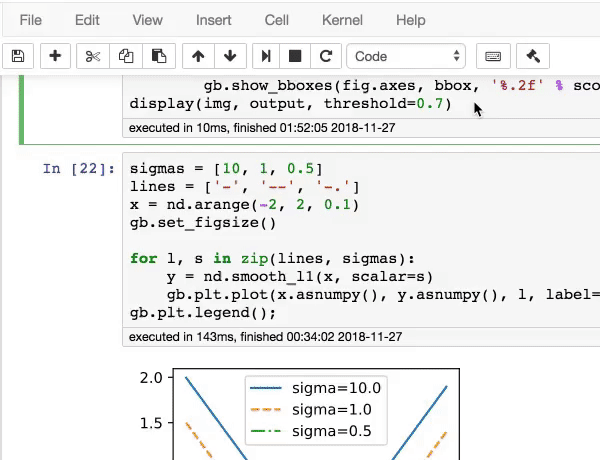公式 + 图示 + 代码

我们不仅结合文字、公式和图示来阐明深度学习里常用的模型和算法，还提供代码来演示如何从零开始实现它们，并使用真实数据来提供一个交互式的学习体验。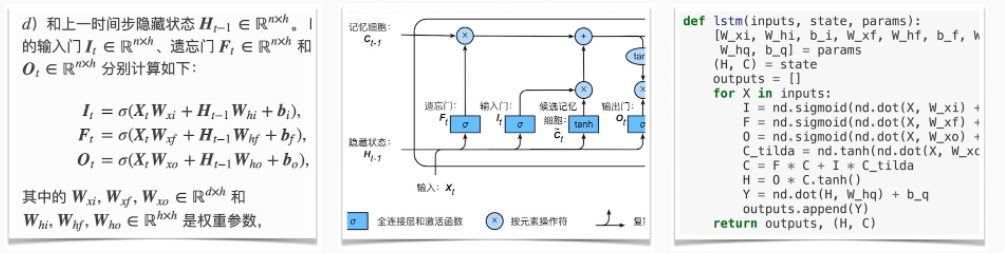活跃社区支持

你可以通过每个章节最后的链接来同社区的数千名小伙伴一起讨论学习。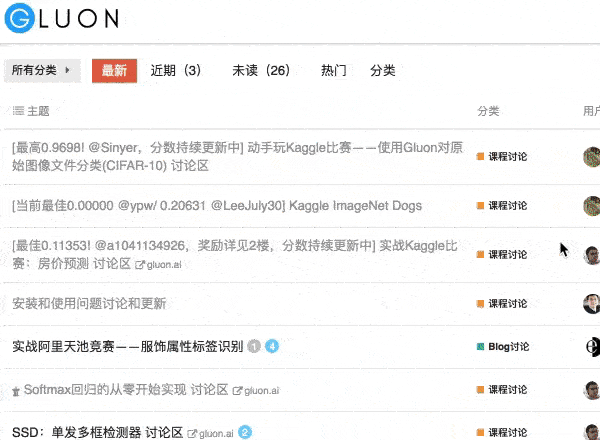被用作教材或参考书

北京大学
复旦大学
哈尔滨工业大学
清华大学
上海财经大学
上海交通大学
浙江大学
中国科学技术大学
Carnegie Mellon University（美国）
Emory University（美国）
Gazi Üniversitesi（土耳其）
Georgia Institute of Technology（美国）
Habib University（巴基斯坦）
Hasso-Plattner-Institut（德国）
Hiroshima University（日本）
Imperial College London（英国）
Indian Institute of Technology Bombay（印度）
Indian Institute of Technology Kanpur（印度）
Indian Institute of Technology Kharagpur（印度）
Indian Institute of Technology Mandi（印度）
Indian Institute of Technology Ropar（印度）
Institut Supérieur De L'electronique Et Du Numérique（法国）
İstanbul Teknik Üniversitesi (土耳其)
King Abdullah University of Science and Technology（沙特阿拉伯）
Kyungpook National University（韩国）
Massachusetts Institute of Technology（美国）
McGill University（加拿大）
National University of Singapore（新加坡）
Nazarbayev University（哈萨克斯坦）
Northeastern University（美国）
Pontificia Universidad Católica de Chile（智利）
Rutgers, The State University of New Jersey（美国）
Sapienza Università di Roma（意大利）
Stanford University（美国）
Technische Universiteit Delft（荷兰）
Texas A&M University（美国）
Universidad Carlos III de Madrid（西班牙）
Universidad Nacional de Colombia Sede Manizales（哥伦比亚）
Universidade Federal de Minas Gerais（巴西）
Università degli Studi di Brescia（意大利）
Universität Heidelberg（德国）
Universitatea de Vest din Timișoara（罗马尼亚）
University of Arkansas（美国）
University of California, Berkeley（美国）
University of California, Los Angeles（美国）
University of California, San Diego（美国）
University of California, Santa Barbara（美国）
University of Illinois at Urbana-Champaign（美国）
University of Maryland（美国）
University of Minnesota, Twin Cities（美国）
University of New Hampshire（美国）
University of North Carolina at Chapel Hill（美国）
University of Pennsylvania（美国）
University of Technology Sydney（澳大利亚）
University of Washington（美国）
University of Waterloo（加拿大）
Universitat Politècnica de Catalunya（西班牙）
Vietnamese-German University（越南）

点击获取下面的资料

1、《动手学深度学习》电子书+源码

2、1467篇CVPR 2020论文（2.69G）

3、CVPR 2020代码开源论文合集

4、ECCV2020论文代码开源的论文合集

展开全文机器学习 人工智能 计算机视觉 tensorflow
• 为研究“低指标突出”问题的内在原因，采用恒温瓦斯放散试验对构造煤的瓦斯放散初期特征进行研究，基于试验结果对K1测定结果偏离真实值的技术原因进行分析，结果表明：采用巴雷尔公式计算K1的理论存在局限性；...
• 第3章 复杂构造叠后深度成像 3.1 逆时深度偏移 3.1.1 方法原理 3.1.2 稳定性条件 3.1.3 数值计算 3.2 四种常用的非Kirchhoff偏移方法 3.2.1 相移加插值（PSPI）法 3.2.2 隐式（ω-x）域有限差分（FD）法 3.2.3 裂步...
• 为有效防治采动影响导致的底板突水事故,采用塑性理论及经验公式分析计算了带压开采工作面底板破坏深度,并进行了突水危险性预测。根据平煤股份十矿24130工作面己18煤层的埋藏特征,通过大量的现场实测和理论分析,绘制...
• 在这项工作中，我们对深度神经网络（DNN）的对抗空间进行了公式化建模，并在精确理解DNN输入和输出之间映射的基础上，引入了一类新的算法来构造对抗样本。在计算机视觉的应用中，我们证明了我们的.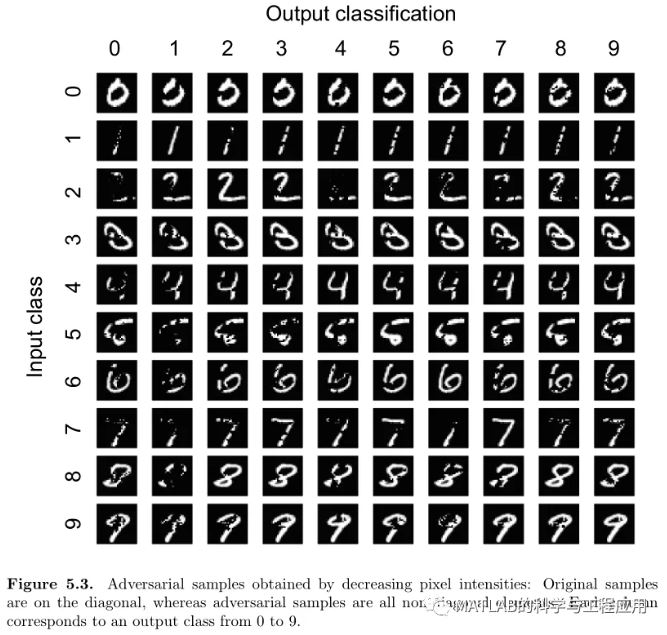本文为美国宾夕法尼亚州立大学（作者：Nicolas Papernot）的硕士论文，共60页。
深度学习利用大数据集和计算效率高的训练算法，在各种机器学习任务中优于其他方法。然而，深度神经网络在训练阶段的不完善，使得它们像其他机器学习技术一样，容易受到对手样本的攻击：对手精心设计输入目的是导致机器学习算法进行错误的分类。
在这项工作中，我们对深度神经网络（DNN）的对抗空间进行了公式化建模，并在精确理解DNN输入和输出之间映射的基础上，引入了一类新的算法来构造对抗样本。在计算机视觉的应用中，我们证明了我们的算法能够可靠地产生由人类被试正确分类的样本，而由DNN在特定目标中错误分类的样本，其对抗成功率为97%，而平均每个样本仅修改4.02%的输入特征。然后，我们通过定义一个硬性度量指标来评估不同样本类对对抗性扰动的脆弱性，最后，通过定义良性输入和目标分类之间距离的预测性度量，我们描述了对抗性样本防御的初步工作。
Deep learning takes advantage of largedatasets and computationally efficient training algorithms to outperform otherapproaches at various machine learning tasks. However, imperfections in thetraining phase of deep neural networks make them, like other machine learningtechniques, vulnerable to adversarial samples: inputs crafted by adversarieswith the intent of causing machine learning algorithms to misclassify. In thiswork, we formalize the space of adversaries against deep neural networks (DNNs)and introduce a novel class of algorithms to craft adversarial samples based ona precise understanding of the mapping between inputs and outputs of DNNs. Inan application to computer vision, we show that our algorithms can reliablyproduce samples correctly classified by human subjects but misclassified inspecific targets by a DNN with a 97% adversarial success rate while onlymodifying on average 4.02% of the input features per sample. We then evaluatethe vulnerability of different sample classes to adversarial perturbations bydefining a hardness measure. Finally, we describe preliminary work outliningdefenses against adversarial samples by defining a predictive measure ofdistance between a benign input and a target classification.

引言
关于深度学习
深度学习中威胁模型的分类
在测试过程中攻击深度神经网络的完善性
攻击效果验证
基于攻击理解的防御机制设计
相关工作
结论

更多精彩文章请关注公众号：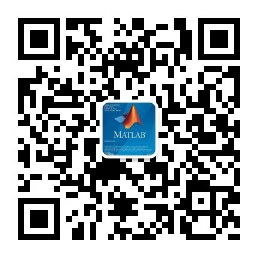展开全文• 公式：其中w1为权重——控制输入信号的重要性；b为偏置——调整神经元被激活的容易程度。 2.“门”的作用：感知机→(门)→应用、计算。 这里的门指：与门、与非门、或门。 它们是具有相同构造的感知机，是线性的，...
• 南力学模型及边界条件建立对原有抗滑桩允许水平承载力的求解公式，解决了国家规范中换算深度<2.4的桩水平承载力的计算。应用本公式评价石城坪滑坡原有抗滑桩的抗滑能力，为岩土工程冶理提供依据。新增了抗滑桩的...
• 决策树 构造决策树的基本想法 随着树深度的增加，节点的熵迅速地下降，熵降低的速度越快越...熵值计算公式 根据历史数据，做某事的概率为2/5，不做某事的概率为3/5 -(2/5)log2(3/5) - (3/5)log2(3/5) -0.4 * -1.321
• 文章目录导入KD树构造构造实例3.2搜索小结 本课程来自深度之眼，部分截图来自课程视频以及李航老师的《统计学习方法》第二版。 公式输入请参考：在线Latex公式 导入 实现k近邻法时，主要考虑的问题是如何对训练数据...
• 在水文地质试验中,对松散层潜水,采取了抽水试验,对两个断裂带,适时的运用观测涌水量和压力水头及涌水试验的方法,并选择适宜的计算公式,较好的完成了该孔的水文地质参数的计算工作,取得了良好的效果。
• 采用测井约束地震反演方法,综合了测井资料和地震资料得到目的层的层速度,预测得到的地层压力在总体上体现出了与深度的密切关系,同时也体现了构造作用等地质因素引起的局部变化,与传统方法根据压力梯度计算结果相比,...
• 本文推导出瞬变电磁场在垂直方向...从视电阻率‐时间剖面图到视电阻率拟深度剖面图（ARPDS）的计算中。然而，视电阻率拟深度剖面图不能 直观地反映出地电构造关系。另外，视电阻率不能直接地反映瞬变电磁场量的大小。
• 总结出相对涌出量随开采深度增大而增大,埋藏越深的煤层一般来说其瓦斯压力、瓦斯含量与相对涌出量越高,瓦斯赋存主要受向斜构造的控制、褶曲构造的控制作用等瓦斯地质规律。并就开采顺序对瓦斯涌出量的影响,采煤工作...
• 机器学习与深度学习的区别 解决过拟合的方法有哪些？什么情况下一定会发生过拟合？...如何构造决策树、计算信息熵和信息增益、说明C4.5 、ID3、 CART的区别及优缺点 Boost,bagging、adaboost、boosti...机器学习
• ACM算法分类={基本算法{枚举✔贪心✔递归和分治✔递推✔构造✔模拟✔搜索{深度优先搜索✔广度优先搜索✔双向搜索启发式搜索记忆化搜索✔计算几何{几何公式✔叉积和点积的运用✔多边形的简单算法✔凸包扫描...
• 我们的运营商通过具有理想特性（各向异性，拓扑感知，轻量级，易于优化）的构造而来，并将其用作传统深度生成架构的构建块，从而展示了最新的成果。与线性Morphable模型和其他图卷积运算符相比，各种3D形状数据集。...Python
• 4.1.6平面多面体与欧拉公式 4.2三维形体的表示 4.2.1多边形表面模型 4.2.2扫描表示 4.2.3构造实体几何法 4.2.4空间位置枚举表示 4.2.5八叉树 4.2.6BSP树 4.2.7OpenGL中的实体模型函数 4.3非规则...
• ## 组合数学及其算法

热门讨论 2011-10-14 20:52:32
3．3 几个重要公式 3．4 错位排列 3．5 有限制的排列 3．6 棋阵多项式 3．7 禁位排列 习 题 第四章 鸽巢原理 4．1 鸽巢原理 4. 2 鸽巢原理的推广形式 4. 3 ramsey数 4．4 ramsey数的性质 4．5 ramsey定理...
• 2.7 欧拉公式 2.8 图论中的一个问题 2.9 格雷码 2.10 在图上寻找无重边的路 2.11 数学平均数和几何平均数定理 2.12 循环不变量：将十进制数转换为二进制数 2.13 常见的错误 2.14 小结 第3章 算法分析 3.1 ...
• 14.3.4 实现构造器和选择器函数 500 14.4 分析表达式 502 14.4.1 语法分析和语法 502 14.4.2 不考虑优先级的语法分析 503 14.4.3 在语法分析器中加入优先级 507 14.5 计算表达式 509 14.6 小结 511 14.7 ...
• python字符串与绘图复习变量None变量参与运算没有赋值的情况不会改变绘制立方体绘制五角星时间计算强化实现贷款计算连续输入多个字符串加法与绘图实现显示线段长度数据函数使用快速查阅函数帮助字符串三种风格字符...python工程师 python爬虫 python python教程 python安装
• 10.3.3 构造函数、析构函数及Winner 函数 308 10.3.4 初始化赢者树 308 10.3.5 重新组织比赛 310 10.4 输者树 311 10.5 应用 312 10.5.1 用最先匹配法求解箱子装载 问题 312 10.5.2 用相邻匹配法求解箱子...
• 10.3.3 构造函数、析构函数及Winner 函数 308 10.3.4 初始化赢者树 308 10.3.5 重新组织比赛 310 10.4 输者树 311 10.5 应用 312 10.5.1 用最先匹配法求解箱子装载 问题 312 10.5.2 用相邻匹配法求解箱子...数据结构
• ③分别选择同一直线上的两条线段的距离值，利用“度量”菜单中的计算命令，依次计算出两者之积④拖动动点，观察规律：相交弦定理。 制表 [制表] 在“度量”菜单中“制表”命令。选择上例中“四条线段的长度”，利用...